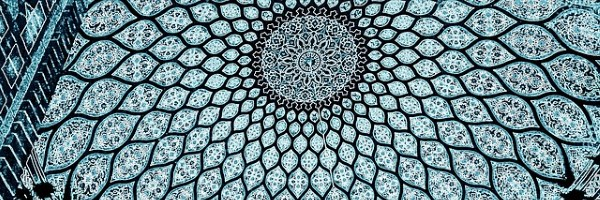# Exploring Number ShapesHave a look at this very short video which illustrates Centred Shapes:

Can you see how Centred Shapes are made?

Now, take a look at this video which shows, using pictures, how centred triangles are related to triangular numbers and square numbers:

Can you convince yourself that this will be the case for all Centred Triangles?

If you would like to read more, take a look at this Word document.

Now take a look at some of these relationships and see what you can discover.

Can create some pictures to show that the relationships are true (a visual proof)?

a/ 3rd Centred Hexagon Number + 1 = 2 of 3rd Centred Triangle Number
(or Nth Centred Hexagon Number + 1 =  2(Nth Centred Triangle Number)

b/ 3rd Centred Square Number – 2nd Triangular Number = 3rd Centred Triangle Number
(or Nth Centred Square Number – (N-1)th Triangular Number = Nth Centred Triangle Number)

c/ 3² + 2² = 3rd Centred Square Number
(or N² + (N-1)² = Nth Centred Square Number)

d/ 2nd Triangular Pyramid Number + 3rd Triangular Pyramid Number = 3rd Square Based Pyramid Number
(or (N-1)th Triangular Pyramid Number + Nth Triangular Pyramid Number = Nth Square Based Pyramid Number)

Once you've had a go at these, you may like to look at Centred Shapes - More to Explore.